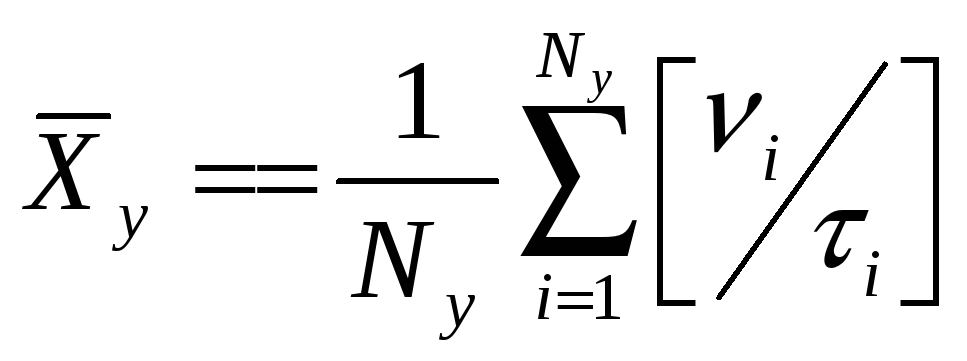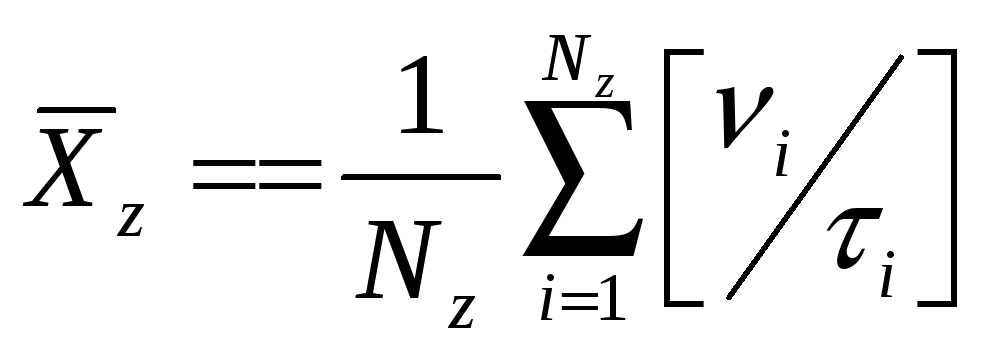# Use of a remotely operated vehicle as awhere, ν = transect count of abalone τ = transect area m2 (“effort on” transect length m x field of view in m) x = 30 – 40m depth strata y = 40 – 50m depth strata z = 50 – 60m depth strata N = Number of samples per strata Second, determine the location population estimate (µ) as, µ= Σ[Xx(φx), Xy(φ y), Xz(φz)] where, φ = total area of sampled location habitat, for each depth stratum (x, y, and z) Third, calculate the 95% confidence intervals of the locations population estimates as: CI= Σ[(x ± 1.96 (σx/√nx), (y ± 1.96 (σy/√n σy),z ± 1.96 (σz/√n σz)] If all locations where suitable white abalone habitat exists are sampled, then the global population (µt), that is all members of the species, can be calculated as, µt = µi + µii + µiii…….. µn and the confidence we have in this estimate can be expressed in confidence intervals as, CIt = CIi + CIii + CIiii……….CIn Share with your friends: